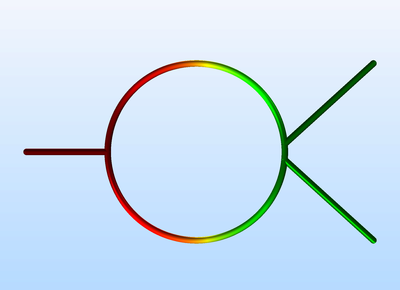Application Gallery

Fast Modeling of a Transmission Line Wilkinson Power Divider

Application ID: 42061

Some conventional three-port power dividers are resistive power dividers and T-junction power dividers. Such dividers are either lossy or not matched to the system reference impedance at all ports. In addition, isolation between two coupled ports is not guaranteed. The Wilkinson power divider outperforms the lossless T-junction divider and the resistive divider and it does not have the previously mentioned issues.

This example model simulates a Wilkinson power divider using the Transmission Line interface in 2D. This approach is very fast compared to solving Maxwell’s equations in 3D. The results present the S-parameters from 1 GHz to 5 GHz and electric potential distribution along the transmission line.This model example illustrates applications of this type that would nominally be built using the following products: